15.03.2021 г.

Quantitative assessment of cupping effect in tomographic images

As you already know, we specialize in image processing here, at Smart Engines. Not so long ago we published an article about binarization of fine-structure tomographic images where we attempted to explain what the x-ray tomography method is about. Today we’ll continue talking about the possible challenges that arise when working with tomographic images, namely the cupping effect artifacts and their quantitative assessment.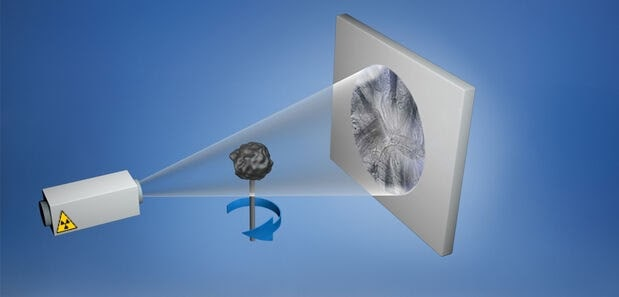Let’s examine a 10 ruble coin. Its photo is shown in Figure 1, on the left. These coins are struck in steel, and then the brass plating is applied to the finished product. That means that the coin consists of two optically homogeneous materials. The first material is a thin layer on the edges, the second material is what the coin itself is made of. The result of tomographic reconstruction of the coin’s cross-section is demonstrated in Figure 1, on the right. The reconstruction was performed using the classic convolution and inverse projection method without correction of the estimation results . Instead of the expected homogenous brightness value inside the coin, we can see the gradual darkening from the edges to the center. And it looks more or less consistent closer to the center of the object. This kind of distortion is known as “the cupping effect”.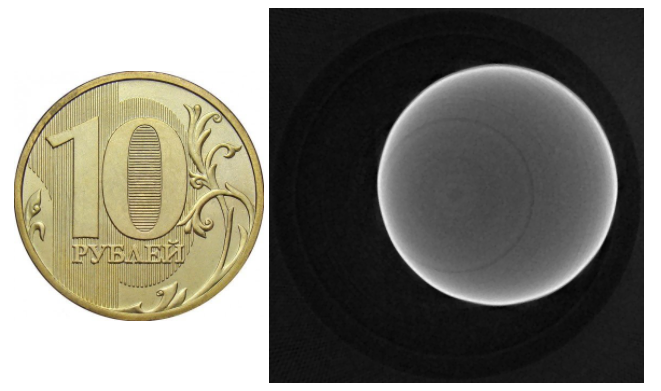Figure 1. The photo of a 10-ruble coin that was used in the tomographic experiment (left), the result of the tomographic reconstruction (right).

Let’s find out what causes the cupping effect in the images produced by the x-ray tomography method. This method could be divided into two stages. The first stage is the collection of 2D images with the help of a tomographic device. We’d like to remind you that during tomographic scanning the object placed on top of the holder rotates and gets x-rayed periodically, at a defined angular step. The radiation that passes through the object is registered by the position-sensitive equipment, and as a result the 2D image of the shadow projection is created. One of the registered images of the coin is shown in Figure 2. As you can see, the coin was placed at an angle. Since the entire object didn’t fit in the detector’s field of view, the final image (Figure 2) was created by pasting together a few separate fragments (with some overlaps). The grey vertical lines around the areas where the fragments were put together are caused by nonuniformity at the edges of the detector. These nonuniformities cause circular-shaped artifacts, but we’ll talk about this problem some other time. We just want to add that for our experiment we used a unique detector  designed for spectral measurements while maintaining the spatial resolution.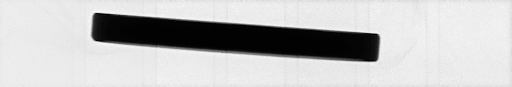Figure 2. A 2D image of the coin registered during the tomographic experiment

However, the x-ray tubes used in medical and industrial tomographs are the broad-band radiation sources. Even if we assume that the main attenuation factor is absorption and ignore the other effects, such as elastic and inelastic scattering, and reemission, the absorption coefficient depends both on the atomic number of the element and the energy of radiation. The lower the energy of radiation, the higher the absorption. With that said, the recorded variables are expressed by a complicated calculation with the added integration over energy. A strong mathematical transformation that would help to make the relationship between the distribution of the absorption coefficients and the number of recorded quant linear, doesn’t exist yet. The use of the popular reconstruction algorithms on the measurement result data which has been standardized for the spectrum integral, causes the cupping effect in the reconstructed images.

The distortions due to the cupping effect in the reconstructed images result in the incorrect interpretations of the results of the tomographic experiment. This problem occurred with the very first version of a tomograph. By the way, the first computed tomograph was a medical device and it was designed by G. N. Hounsfield in 1969 . In medical tomographic images, the cupping effect manifests itself when the bone tissue is scanned, because the bone tissue attenuates radiation more than any other tissues. This effect was discovered and described by R. A. Brooks and G. Di Chiro in 1976. Since then there were many attempts to eliminate or at least to suppress the circular distortions in the tomographic images.

Today there are a great number of approaches to tackling this problem. These approaches can be divided into two groups: hardware based and software based solutions. The hardware based solutions include the methods of spectral composition change of probing radiation. For instance, an x-ray radiation filter can be installed in the optical path of the tomograph, between the x-ray tube and the sample, and it will attenuate the low-energy part of the spectrum. The software based solutions rely on the algorithmic decisions for image processing. Both recorded and reconstructed images can be processed here.

Each method has its own specific conditions for their applicability and, oftentimes, they have one or several adjustable parameters. For example, both the material and the thickness of the x-ray radiation filters can be modified in the hardware based solutions.

In order to determine the most appropriate method for suppression of the cupping effect and the option settings for the chosen method, the visual estimation of the results based on the expert opinions is often used. And it consists of the analysis of the brightness profile of the reconstructed image, as shown in Figure 3.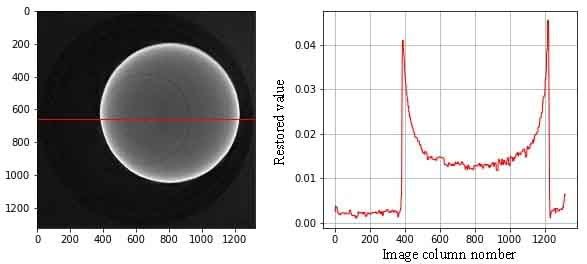Figure 3. The reconstructed image (left). The image side-view at the spot marked by the line in the image on the left (right).

These profiles are created for a few reconstructed images of the same sample while applying various methods for reducing the circular artefacts. The experts decide which method works best for suppressing distortions based on their visual estimation. However, this approach is subjective and ineffective because slight changes would be hardly perceptible to a man’s eyes. Therefore, there is a need to create a method for the quantitative assessment of circular artefacts in the reconstructed images. And that’s exactly what we did.

When we were working on this method, we took a note of the fact that the circular distortions occur inside optically-dense objects in the form of gradual darkening of the object from the edges to the center and stable values in the central region of the object. Therefore, the problem of the quantitative assessment of the cupping effect consists of the assessment of distortions of the individual objects in the reconstructed image. The principal idea of this method is the introduction of the Euclidean distance map. The map is constructed by applying the distance transform  to a binary image. Two types of pixels in a binary image identify the objects and the background. The transformation result is a grayscale image with integer values. These values equal an Euclidean distance from each pixel to the closest boundary pixel. There is a binary image shown in Figure 4, on the left, and the image which is a result of the distance transform, i.e. the Euclidean distance map, on the right.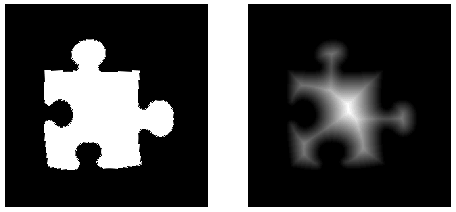Figure 4. The binary image of the object (left). The Euclidean distance map of the object (right).

Based on the Euclidean distance map of the object, the estimate of the circular distortions is calculated as the variation in intensity between the boundary pixels and the central ones. As a result, we’ll get a value that indicates the degree of the cupping effect intensity for each object. If there are several objects present in the reconstructed image, the average value is calculated and it’s considered to be the final answer.

Using the framework of the above-mentioned method, we’ll be able to build an algorithm which has a two-dimensional reconstructed image and a mask of this image with pre-labeled objects as the input parameters. The output of the algorithm would be a quantitative measure of the cupping effect. The algorithm consists of the following steps:

— The image mask is divided according to its objects.
— The Euclidean distance map is calculated for each object.
— Based on the calculated distance map, the central area of an object is determined. It contains the pixels with the distance to the edges of the object being more than 80% of the maximum distance.
— The stable value is calculated as the average value for the central area of the image.
— Based on the calculated distance map, the peripheral area is determined. It contains the pixels for which the distance to the edges of the object is not more than 20% of the maximum distance.
— The deviation of the intensity value from the stable value is calculated for each pixel in the peripheral area. The deviation results are averaged. — The values calculated for each object of the image are totted up and normalized to their total number. The generated value will be the final identifier for the artifact intensity.

We published the algorithm in the paper «Method for numeric estimation of Cupping effect on CT images» which was presented at the international conference ICMV in November, 2019, Amsterdam, Netherlands .

In order to demonstrate the performance of the proposed method, let’s turn to the image of the coin again. The numerical value for the cupping effect intensity in the original image (Figure 5, on the left) was 0.50. Then the reconstruction method with the input data adjusted for the generation model of the tomographic projections in polychromatic conditions was applied to the image. And, as a result, the visual representation of the reconstructed image improved (Figure 5, on the right), and the intensity value decreased to 0.18.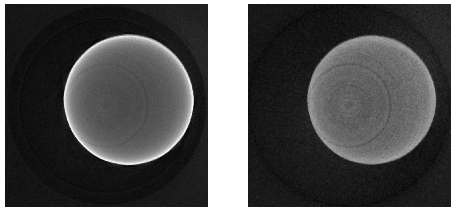Figure 5. The reconstructed coin before the method for suppression of the circular artefacts was applied to it (left). After applying the method (right).

Today we reviewed the problem of circular artefacts in the tomographic images. We attempted to explain what can possibly cause these distortions and to show the need for the method for the quantitative assessment of distortions. We built the algorithm for the quantitative assessment of the cupping effect intensity which has a number of advantages. First of all, the estimate calculated by the algorithm doesn’t depend on the number of objects and their sizes. Second, the quantitative assessment doesn’t depend on the optical density of the image in its entirety and its objects. Therefore, the designed algorithm can be used for automatic processing of the results of the tomographic experiment. It can also be used for selection of the parameters of the methods for suppression of the circular artifacts.

Bibliography
 A. S. Ingacheva and M. V. Chukalina, “Polychromatic CT Data Improvement with One-Parameter Power Correction,” Mathematical Problems in Engineering, vol. 2019, 1405365, 2019, DOI: 10.1155/2019/1405365.
 Kozhevnikov, D. and Solyanskiy P., 2019. Equalization of Medipix family detector energy thresholds using X-ray tube spectrum high energy cut-off. Journal of Instrumentation, vol. 14, num. 1, T01006.
 Появление компьютерных томографов.
 Distance transform (https://en.wikipedia.org/wiki/Distance_transform).
 A. Ingacheva, M. Chukalina, A. Buzmakov and D. Nikolaev, “Method for numeric estimation of Cupping effect on CT images,” ICMV 2019, 11433 ed., Wolfgang Osten, Dmitry Nikolaev, Jianhong Zhou, Ed., SPIE, Jan. 2020, vol. 11433, ISSN 0277-786X, ISBN 978-15-10636-43-9, vol. 11433, 1143331, 2020, DOI: 10.1117/12.2557167.